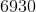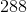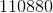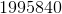## Example Questions

### Example Question #5 : Integers

Find the greatest common factor of 16 and 24.

16

4

2

8

8

Explanation:

First, find all of the factors of each number. Factors are the numbers that, like 16 and 24, can evenly be divided. The factors of 16 are 1, 2, 4, 8, 16. The factors of 24 are 1, 2, 3, 4, 6, 8, 12, 24.

Now, to find the greatest common factor, we find the largest number that is on both lists. This number is 8.

### Example Question #6 : Integers

What is the greatest common factor of 18 and 24?

24

3

18

9

6

6

Explanation:

The greatest common factor is the greatest factor that divides both numbers. To find the greatest common factor, first list the prime factors of each number.

18 = 2 * 3 * 3

24 = 2 * 2 * 2 * 3

18 and 24 share one 2 and one 3 in common.  We multiply them to get the GCF, so 2 * 3 = 6 is the GCF of 18 and 24.

### Example Question #7 : Integers

What is the greatest common factor ofand?Explanation:

To make things easier, note 6930 is divisible by 30:

6930 = 231 * 30 = 3 * 77 * 3 * 2 * 5 = 3 * 7 * 11 * 3 * 2 * 5 = 2 * 32 * 5 * 7 * 11

288 = 2 * 144 = 2 * 12 * 12 = 2 * 2 * 2 * 3 * 2 * 2 * 3 = 25 * 32

Consider each of these "next to each other":

25 * 32

2  * 32 * 5 * 7 * 11

Each shares factors of 2 and 3.  In the case of 2, they share 1 factor.  In the case of 3, they share 2 factors.  Therefore, their greatest common factor is: 2 * 32 = 2 * 9 = 18

Tired of practice problems?

Try live online GRE prep today.